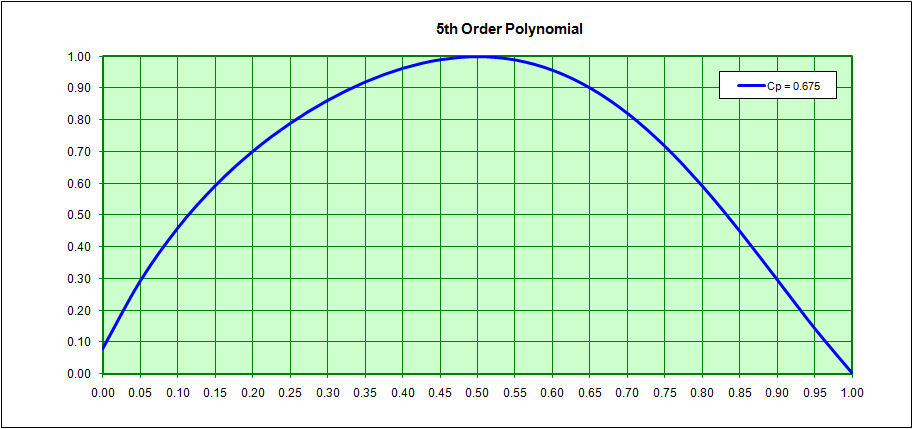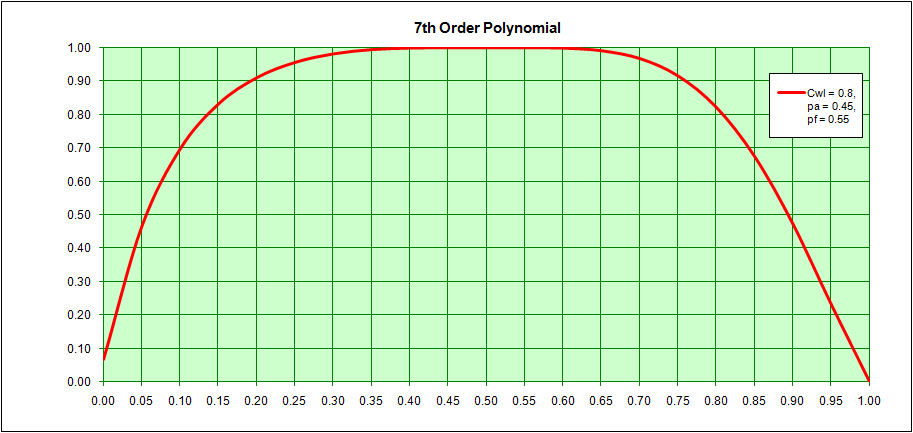The Istanbul Technical University Method Here is another method, that I believe is from the Istanbul Technical University, in which a ship's entire waterline or sectional area curve can be treated as a single 5th order polynomial for ship's with no parallel midbody or as a 7th order polynomial for ships with parallel midbody. Specifically, for waterlines and sectional area curves without parallel midbody;      y = a + bx + cx^2 + dx^3 +ex^4 + fx^5 Where x = 0  is the transom and x = 1 is the bow Here, for the sectional area curve, the boundary conditions are; @ x = 0, y = the Transom Area Coefficient (At/Ax) @ x = 1, y = 0 (assuming the ship does not have a bulbous bow) @ x = 0.5, y = 1 (assuming that the midship section is equal to the section of max area) @ x = 0.5, y' = 0 (as above) S y dx = Cp S yx dx = Cp ( 0.5 - LCB / 100 ) For the Design Waterline, these boundary conditions are; @ x = 0, y = the Transom Beam Coefficient (Bt/Bx) @ x = 1, y = 0 (assuming the ship does not have a bulbous bow) @ x = 0.5, y = 1 (assuming that the midship section is equal to the section of max beam) @ x = 0.5, y' = 0 (as above) S y dx = Cwl S yx dx = Cwl ( 0.5 - LCF / 100 ) For ships with parallel midbody     y = a + bx + cx^2 + dx^3 +ex^4 + fx^5 + gx^6 + hx^7 Here, for the sectional area curve, two additional points are defined as     pf = the forward extent of the parallel midbody     pa = the aft extent of the parallel midbody The boundary conditions are; @ x = 0, y = the Transom Area Coefficient (At/Ax) @ x = 1, y = 0 (assuming the ship does not have a bulbous bow) @ x = pf, y = 1 (assuming that throughout the extent of the parallel midbody sectional area = maximum area) @ x = pa, y = 1 (as above) @ x = pf, y' = 0 (as above) @ x = pa, y' = 0 (as above) S y dx = Cp S yx dx = Cp ( 0.5 - LCB / 100 ) For the Design Waterline, these boundary conditions are; @ x = 0, y = the Transom Beam Coefficient (Bt/Bx) @ x = 1, y = 0 (assuming the ship does not have a bulbous bow) @ x = pf, y = 1 (assuming that throughout the extent of the parallel midbody sectional area = max beam) @ x = pa, y = 1 (as above) @ x = pf, y' = 0 (as above) @ x = pa, y' = 0 (as above) S y dx = Cwl S yx dx = Cwl ( 0.5 - LCF / 100 ) Using the Matrix ("Array") capabilities of a Spreadsheet Program like Excel it is fairly easy to solve for the coefficients in the original polynomials. A sample of the 5th order and 7th order polynomials is shown below.Home